Miscellaneous

Chapter 6 Class 11 Permutations and Combinations
Serial order wise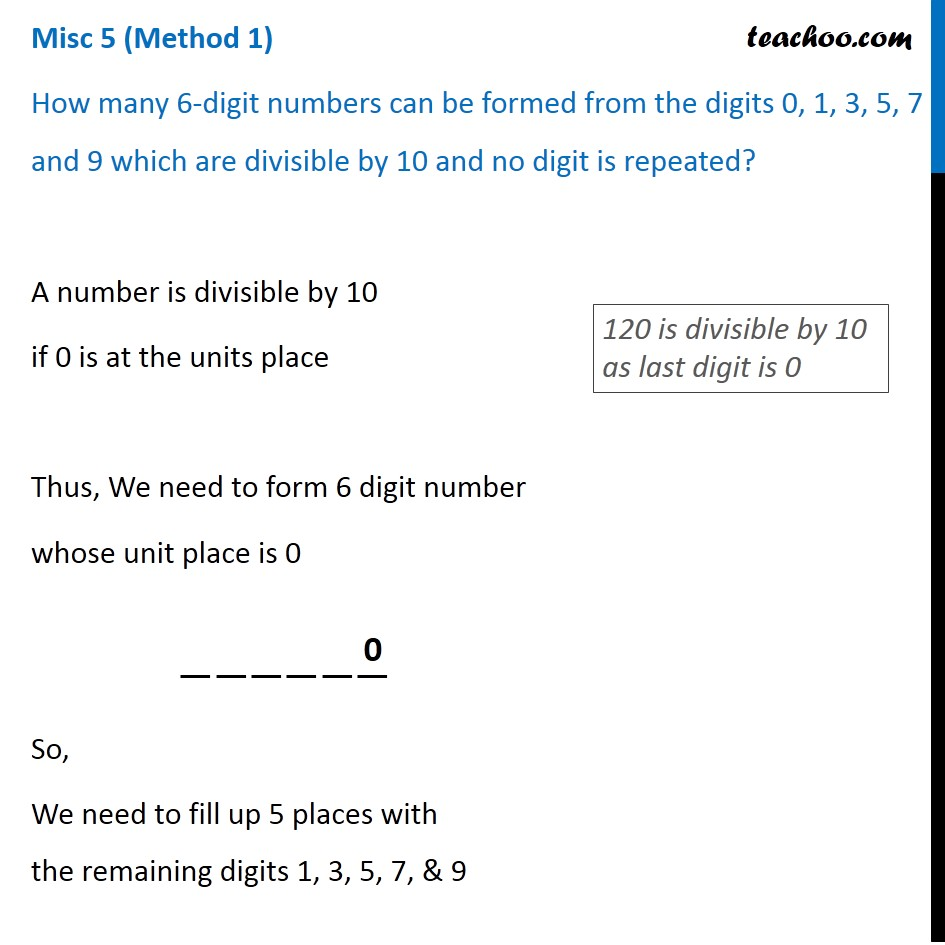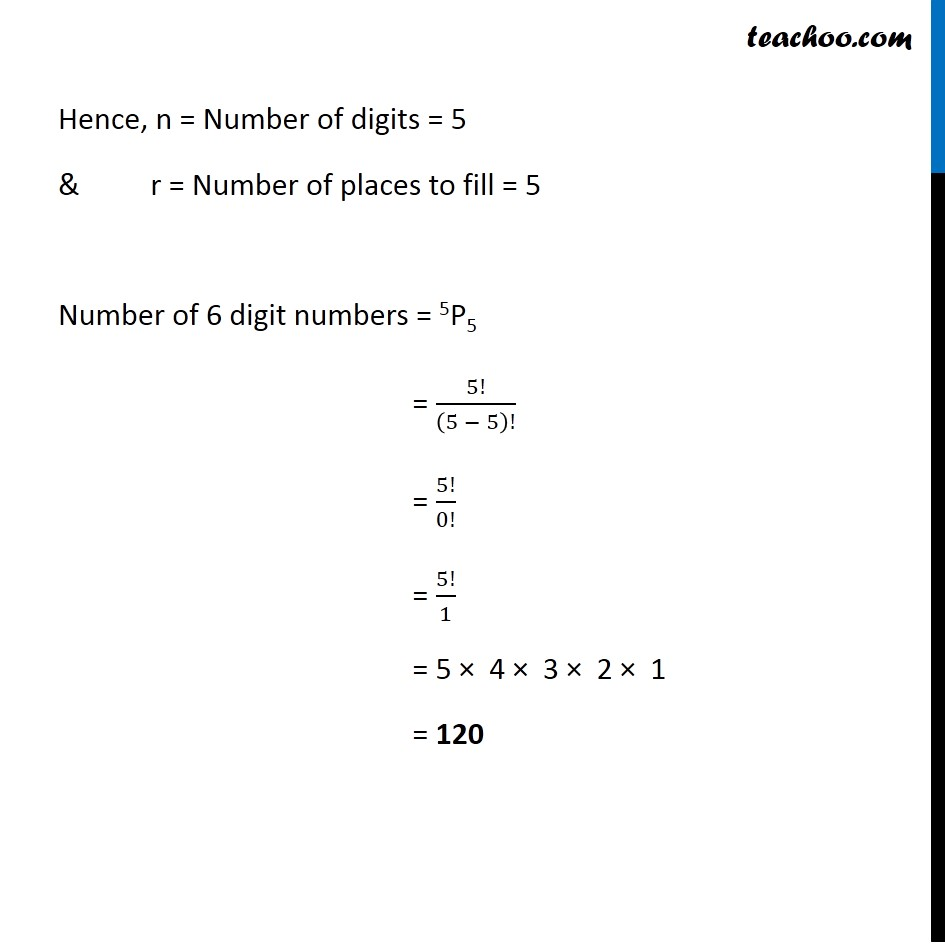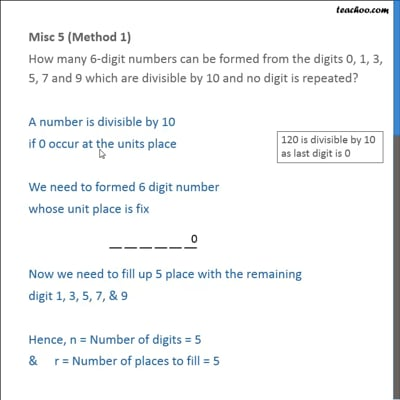This video is only available for Teachoo black users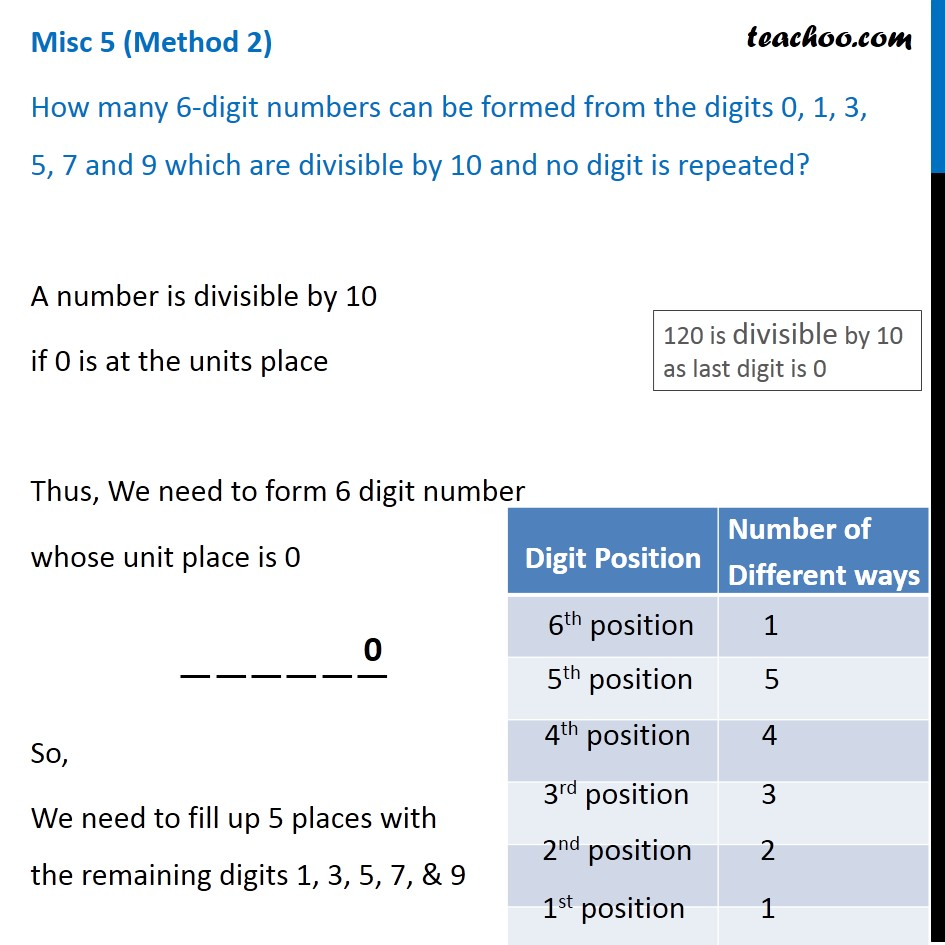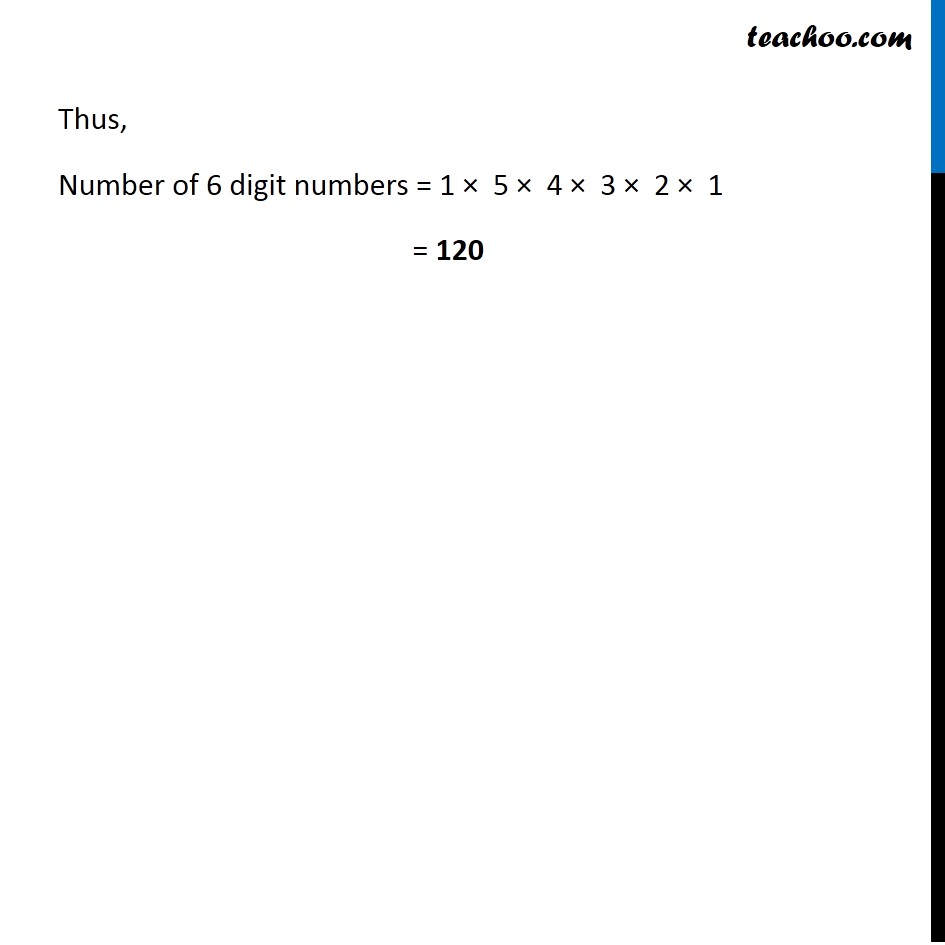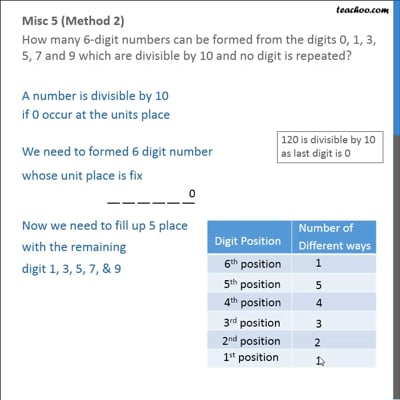This video is only available for Teachoo black users

Learn in your speed, with individual attention - Teachoo Maths 1-on-1 Class

### Transcript

Misc 5 (Method 1) How many 6-digit numbers can be formed from the digits 0, 1, 3, 5, 7 and 9 which are divisible by 10 and no digit is repeated? A number is divisible by 10 if 0 is at the units place Thus, We need to form 6 digit number whose unit place is 0 So, We need to fill up 5 places with the remaining digits 1, 3, 5, 7, & 9 120 is divisible by 10 as last digit is 0 Hence, n = Number of digits = 5 & r = Number of places to fill = 5 Number of 6 digit numbers = 5P5 = 5!/(5 − 5)! = 5!/0! = 5!/1 = 5 × 4 × 3 × 2 × 1 = 120 Misc 5 (Method 2) How many 6-digit numbers can be formed from the digits 0, 1, 3, 5, 7 and 9 which are divisible by 10 and no digit is repeated? A number is divisible by 10 if 0 is at the units place Thus, We need to form 6 digit number whose unit place is 0 So, We need to fill up 5 places with the remaining digits 1, 3, 5, 7, & 9 120 is divisible by 10 as last digit is 0 Thus, Number of 6 digit numbers = 1 × 5 × 4 × 3 × 2 × 1 = 120